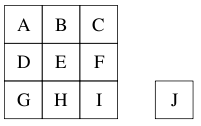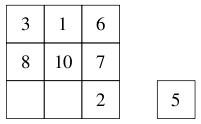# Moduic Squares

Time Limit : 8 sec, Memory Limit : 131072 KB

# Problem A: Moduic Squares

Have you ever heard of Moduic Squares? They are like 3 × 3 Magic Squares, but each of them has one extra cell called a moduic cell. Hence a Moduic Square has the following form.Figure 1: A Moduic Square

Each of cells labeled from A to J contains one number from 1 to 10, where no two cells contain the same number. The sums of three numbers in the same rows, in the same columns, and in the diagonals in the 3 × 3 cells must be congruent modulo the number in the moduic cell. Here is an example Moduic Square:Figure 2: An example Moduic Square

You can easily see that all the sums are congruent to 0 modulo 5.

Now, we can consider interesting puzzles in which we complete Moduic Squares with partially filled cells. For example, we can complete the following square by filling 4 into the empty cell on the left and 9 on the right. Alternatively, we can also complete the square by filling 9 on the left and 4 on the right. So this puzzle has two possible solutions.Figure 3: A Moduic Square as a puzzle

Your task is to write a program that determines the number of solutions for each given puzzle.

## Input

The input contains multiple test cases. Each test case is specified on a single line made of 10 integers that represent cells A, B, C, D, E, F, G, H, I, and J as shown in the first figure of the problem statement. Positive integer means that the corresponding cell is already filled with the integer. Zero means that the corresponding cell is left empty.

The end of input is identified with a line containing ten of -1’s. This is not part of test cases.

## Output

For each test case, output a single integer indicating the number of solutions on a line. There may be cases with no solutions, in which you should output 0 as the number.

## Sample Input

```3 1 6 8 10 7 0 0 2 5
0 0 0 0 0 0 0 0 0 1
-1 -1 -1 -1 -1 -1 -1 -1 -1 -1
```

## Output for the Sample Input

```2
362880
```

Source: ACM-ICPC Japan Alumni Group Summer Camp 2006 , Day 2, Tokyo, Japan, 2006-09-23
http://jag-icpc.org/